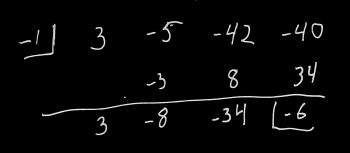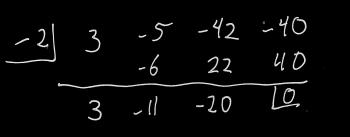Games
Problems
Go Pro!

Wesley writes, "Is there a better way of factoring polynomials other than guess and check? For example: factoring 21x- 2x2 + 4x - 1, you probably will have to guess and check what goes in the parentheses. It could be (3x - something)(7x + something)(x - something)..., or, it could be something entirely different. Aside from the limited equations like difference of squares, or sum/difference of cubes, I would like to know if it is possible to not have to guess and check. If you do not know of a solution, thank you anyways for your time."

Hi Wesley, In addition to the rules that you mentioned, you can find a few more rules here, including sum/difference of odd powers, sum of even powers, grouping, and something I like to call aggressive grouping.

If none of those help, another tool that might help you out is something called the Rational Root Theorem. The rational root theorem says that if a rational zero to a polynomial exists, then written in simplest form p/q, p is a factor of the constant, and q is a constant of the highest order coefficient.

How does this help in factoring? Well, if p/q is a zero of the polynomial, then (qx - p) is a factor.

It's important to note that the statement above has a big "if" in it. It's possible that no rational roots exist. But if they do exist, this rule helps us out in our trial-and-error search by eliminating possibilities.

Let's take an example: 3x3 - 5x2 - 42x - 40 = 0.

The rational root theorem tells us that if this has rational roots, they are of the form p/q, where p is a factor of 40, and q is a factor of 3. Now, this still gives us a LOT of possibilities to consider, because p could be 1, 2, 4, 5, 8, 10, 20, or 40 (plus the opposites of all of those), and q could be either 1 or 3 (or their opposites).

So let's start with something that's not a fraction: Let's try 1, and see what happens. I'm going to do synthetic division on this. If you don't know how synthetic division works, you'll want to take a look at this explanation: How to do synthetic division.This left us with a remainder of -6, and we're looking for something that gives us a remainder of 0. Let's try 2:Ah ha! That did it. That means we know that -2 is a root, or (x - (-2)) is a factor.

The next bit of good news is that we can use the values in our synthetic division to reduce the polynomial by the factor we just found: our polynomial can be rewritten as (x + 2)(3x2 - 11x - 20) (note the numbers at the bottom of our synthetic division - they are the coefficients of the reduced polynomial). To find our next factors we can use the techniques that we're familiar with on the factor 3x2 - 11x - 20, or we can continue doing synthetic division.

Of course, I cheated; I created a polynomial which I knew could be factored, but there's no guarantee that a given polynomial is factorable over real numbers, let alone rational numbers! Your polynomial, for example, has only one real root, and that one real root is not rational. If you had tried the rational root theorem on this, you would have tried 1, 1/3, 1/7, 1/21, and the opposites of all of those, only to find that none of them work. The good news is that because of the rational root theorem, you KNOW that you're done looking for rational roots.

So there are a few more tools for your arsenal; I hope something here was helpful!# Blogs on This SiteReviews and book lists - books we love!The site administrator fields questions from visitors.Like us on Facebook to get updates about new resources